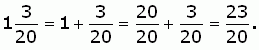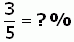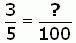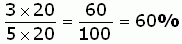SEARCH HOMEMath Central Quandaries & QueriesQuestion from Anita, a student: How do I turn 1 3/20 to a percent?We have two responses for you

Hi Anita.

A "percent" is really just a fraction. Literally, it means "per hundred", and "per" means division. To convert fractions to percentages then, you just need to convert the denominator (bottom) of the fraction into 100.

In your question, you have a "mixed fraction", so first you need to convert this to an "improper fraction":Now you have to convert the fraction 23/20 into a percentage. Because the numerator (top part) is larger than the denominator, you know it will be more than 100%. That's okay, percentage can be larger than 100 in many situations. For instance I usually have to pay 112% of what I see on a price tag, because an extra 12% is added to the price at the checkout for sales tax.

Here's an example of converting a fraction to a percentage. This technique will work with any fraction:

Sample problem:
Convert 3/5 to a percentage.Since percentages are really just fractions with the denominator of 100, we can saySo to turn the denominator from a 5 to a 100, what do we have to multiply by? Well 5×20=100, so we can multiply the top and bottom of the fraction by 20:Now we have the answer: 60 %.

You can use this method on any fraction, Anita. Try it for 23/20 now.

Hope this helps,
Stephen.

Anita,

Remember, a percent is a fraction written over 100. So, what is equivalent to 3/20 or,

1 3/20 = 23/20= ?/100

(What did you do to 20 to make it 100? Do the same to 3)

Check this example:

5/50 = 10/100

because I multiplied 50 by 2(=100), I must also multiply 5 by 2(=10).

Good luck!
MelanieMath Central is supported by the University of Regina and The Pacific Institute for the Mathematical Sciences.